# TS 10th Class Physical Science Important Questions Chapter 6 Structure of Atom

These TS 10th Class Physical Science Chapter Wise Important Questions Chapter 6 Structure of Atom will help the students to improve their time and approach.

## TS 10th Class Physical Science Important Questions Chapter 6 Structure of Atom

1 Mark Questions

Question 1.
What is the speed of the light?
The speed of light (c) ¡s 3 x 108 ms-1.

Question 2.
Define wavelength.
The wavelength of the wave ¡s the distance from one wave peak to the next.

Question 3.
Define frequency (v).
The frequency (v) of a wave Is simply the number of wave peaks that pass through a given point In unit time.
v=1/λ (or) c = vλ, where ‘c’ is the speed of light in vacuum.

Question 4.
What is electromagnetic spectrum?
electromagnetic waves have a wide variety of frequencies. The entire range of electromagnetic wave frequencies Is known as the electromagnetic spectrum.Question 5.
What is visible spectrum? What Is its wavelength range?
The range of wavelengths covering red colour to violet colour is called the visible spectrum. It can be seen by naked eye. Its wavelength range is from 400 nm to 700 nm.

Question 6.
What is the significance of Plancks’ proposal?
The significance of PlanKc’s proposal Is that electromagnetic energy can be gained or lost in discrete values and not in a continuous manner.

Question 7.
What is the use of line spectra?
The lines in atomic spectra can be used to identify unknown atoms, just like fingerprints are used to Identify Individual people.

Question 8.
What do you mean by ground state?
The lowest energy state of the electron is known as ground state.

Question 9.
What happens when an electron gains energy?
When an electron gains energy it moves to a higher energy level, known as excited state.

Question 10.
What are the failures of Bohr’s model of atom?

1. Bohr’s model failed to account for splitting of line spectra.
2. This model failed to account for the atomic spectra of atoms possessing more than one electron.

Question 11.
What is Sommerfeld’s contribution for the structure of atom?
In an attempt to account for the splitting of line spectra. Sommerfeld modified Bohr’s atomic model by adding elliptical orbits.

Question 12.
What is an orbital?
The region of space around the nucleus where the probability of finding the electron is high is called an orbital.

Question 13.
What are quantum numbers?
Each electron in an atom Is defined by a set of three numbers called quantum numbers. These numbers indicate the probability of finding the electron In the space around the nucleus.

Question 14.
What Information do the quantum numbers provide?
The quantum numbers describe the space around the nucleus where the electrons can be found and also their energies.

Question 15.
How many electrons can occupy an orbital?
An orbital can hold only two electrons.

Question 16.
When are electromagnetic waves produced?
Electromagnetic waves are produced when an electric charge vibrates.

Question 17.
What happens when an object is suitably excited by heating?
Light is emitted by the object.

Question 18.
What is an electronic configuration?
The distribution of electrons In shells, sub-shells and orbitals In an atom is known as electronic configuration.

Question 19.
State the Paull’s exclusion principle.
According to Pauli’s exclusion principle, no two electrons of the same atom can have all four quantum numbers the same.

Question 20.
Write the Aufbau principle.
In the ground state, the electronic configuration can be built up by placing electrons in the lowest available orbitals until the total number of electrons added is equal to the atomic number.Question 21.
What is Hund’s rule?
Hund’s rule: According to this rule, electron pairing In orbital’, starts only when all available empty orbitals of the same energy are singly occupied.

Question 22.
Give an example for continuous spectrum.
Rainbow.

Question 23.
Which is example for line spectrum?
The atomic spectrum of hydrogen atom.

Question 24.
Which model explains fine spectrum of atom?
Bohr – Sommerfeld model.

Question 25.
How wavelength and velocity of light related?
c = vλ
where c = velocity of light
v = frequency of light
λ = wavelength of light.

Question 26.
What is meant by Aufbau?
The German word Aufbau means building up.

Question 27.
Which group elements are called Noble gases?
VIII A group or 18th group elements are called inert gases (or) Noble gases.

Question 28.
Which elements are examples for Noble gases?
helium (tie), Neon (Ne), Argon (Ar), Krypton (Kr), Xenon (Xe) and Radon (Rn) are examples for Noble gases.Question 29.
Which elements are highly stable?
Noble gases are highly stable.

Question 30.
Which element has duplet configuration?
The inert gas Helium (1s2).

Question 31.
What Is the general electronic configuration of Noble gases?
ns2 np6

Question 32.
Why is spin quantum number Introduced?
When we observe spectrum of yellow light by using high-resolution spectroscope It has very closely spaced doublet. Similar patterns are shown by Alkali and Alkaline earth metals. In order to account this, spin quantum number is introduced.

Question 33.
Write the set of quantum numbers for the electrons In a 3p0. orbital.

 Orbital n l m1 ms First electron 3 2 0 +1/2 Second electron 3 2 0 -1/2

Question 34.
What is the difference between an orbit and orbital?
An orbit is a well-defined path of electron that revolves around the nucleus. An orbital Is the space around the nucleus, where the probability of finding electrons Is maximum.

Question 35.
Write the set of quantum numbers for the added electron of oxygen atom.
Configuration of oxygen is Is22s22p4.
The added electron is the 4th in the 2p.
The set of quantum numbers(2, 1, -1, 1/2)

Question 36.
What are the factors which influence electromagnetic energy?
Electromagnetic energy depends on two factors.
They are :

1. wavelength
2. frequency

Question 37.
Write the four quantum numbers for the differentiating electrons of lithium (Li) atom.
The electronic configuration of lithium is 1s2 2s1. So differentiating electron enters into 2s. The values of four quantum numbers as given below.

 n l m1 m1s 2 0 0 +½or-½

Question 38.
Write four quantum numbers for 2p1 electrons.
The four quantum numbers for 2p are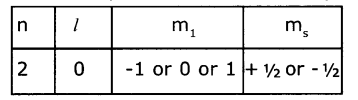Question 39.
If n=3, mention the orbitals present in shell and write maximum number of electrons In the shell.

1. If n=3, the shell Is M shell. OrbitaIs present In this shell are s, p and d.
2. Maximum number of electrons present in this shell are 18(2n2= 2 x 32=18)

Question 40.
How many maximum numbers of electrons that can be accommodated In an l sub-shell?
l subshell has 3 orbitals, Each orbital accommodates 2 electrons. So 6 electrons can be filled in I sub shell.

Question 41.
How many maximum number of electrons can be accommodated In ‘d’ orbital? (AS1)
d sub shell has 5 orbitaIs. So 10 electrons can be filled in d subshell.

Question 42.
What is Zeeman effect and Stark effect?

• Zeeman effect: The splitting up of spectral lines in the presence of strong external magnetic field is called Zeeman effect.
• Stark effect: The splitting up of spectral lines in presence of strong electrical field is called Stark effect.

Question 43.
Write the symbol of the outermost shell of magnesium (Z=12) atom. How many electrons are present In the outermost shell of magnesium?
The symbol Is ‘M’. The electrons present in outermost shell are 2.

Question 44.
The four quantum number values of the 21st electron of scandium (Sc) are given In the following table.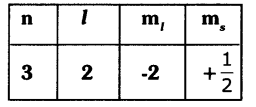Write the values of the four quantum numbers for the 20th electron of scandium (Sc) in the form of the table.
n=4, l=0, m1 = 0, ms = –$$\frac{1}{2}$$

2 Marks Questions

Question 1.
The electronic configuration of an atom is as follows 1S22S22p2.
a) Which element’s atom is it?
b) Which orbital Is the last electron In?
c) When excited what could be the number of lone / sIngle electrons In this atom? (AS1)
d)What is the value of principal quantum numbers of two electrons in the first box?
Given electronic configuration of atom is 1S22S22p2.
a)The element is carbon.
b)The valence electron enters into 2p orbital.
c) In excited state the electron in 2S orbital enters into 2P orbital. So it has 4 unpaired electrons.
d)The value of principal quantum number is 1.

Question 2.
How do the vibrating electric and magnetic fields around the charge become a wave that travel through space?
A vibrating electric charge creates a change In the electric field. The change in electric field creates a change In magnetic field. This process continues, with both the created fields being perpendicular to each other and at right angles to the direction of propagation of the wave.Question 3.
What are the characteristics of electromagnetic waves?

1. electromagnetic waves can be described by means of vibrating electrical and magnetic fields which are mutually perpendicular to each other.
2. The nature of these waves is transverse. These waves travel with a speed equal to the speed of light In vacuum.

Question 4.
Fill the following table and write the rule which you used in tilling the table.

 l 0 1 2 3 Sub-shell s P d f Number of orbitals

 l 0 1 2 3 Sub-shell s p d f Number of orbitaIs 1 3 5 7

The number of orbitals for a sub-shell Is given by (2l + 1) rule.

Question 5.
Write the differences between orbit and orbital.

 Orbit Orbital 1. The path of an electron which revolving around the nucleus in an orbit. 1. The probability of finding electron around the nucleus in an orbital. 2. Orbits are represented by K, L, M, N or 1,2,3,4 2. Orbitals are represented by s, p, d, f, g. 3. Orbits are circular/ elliptical in shape. 3. Orbitals have different shapes like spherical (s), dumbell (p), double dumbell. (d) etc. 4. The maximum number of electrons in any orbit is 2n2. 4. The maximum number of electrons in an orbitai is 2.

Question 6.
The electron enters into 4s orbital after filling 3p orbital but not Into 3d. Explain the reason.
As per Aufbau principle, electron enters into the orbital whose (n +l) value ¡s less.
The (n +l) values for 4s and 3d are
4s = 4+0 = 4
3d = 3+2 = 5
Here the (n+1) value of 4s < 3d. Hence electron enters Into 4s orbital after filling ‘3p instead of’3d.

Question 7.
Give the equation which gives electromagnetic energy (light) can have only certain discrete energy values.
E = hv E=Energyoflight
h = Planck’s constant = 6.625 x 10-27 erg sec or 6.625 x 10-34 Joule-sec
This equation Is called Planck’s equation.

Question 8.
Explain Paulis Exclusion principle with an example.
Pauil’s exclusion principle: According to Paull’s exclusion principle, no two electrons of the same atom can have all four quantum numbers are same.
Eg: He (Z = 2)
The electronic configuration is 1s2,i.e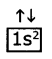The set of four quantum numbers Is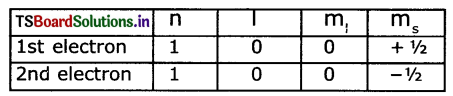Question 9.
Explain Aufbau principle with an example.
Aufbau Principle: Orbitais are filled in the order of increasing energy.
Two general rules that help us to predict electronic configuration.
1. Electrons are assigned to orbitals in order of increasing value of (n + l)
2. For sub-shells with the same value of (n + l), electrons are assigned first to the sub-shell with lower ‘n
Ascending order of energies of various atomic orbitals is given below :
Is<2s<2p<3s<3p<4s<3d<4p<5s<4d<Sp<6s<4f<5d< 6p<7s< 5f<6d<7p<8s.

Question 10.
Explain Hund’s rule with an example.
Hund’s Rule: According to this rule electron pairing In orbitals starts only when all available empty orbitals of the same energy are singly occupied.
Eg :
1. The configuration of carbon atom (Z = 6) is 1s2 2s2 2p2.
2. The first four electrons go into the is and 2s orbitals.
3. The next two electrons go into separate 2p orbitalis, with both electrons having the same in.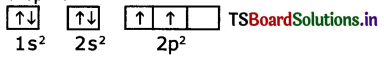4. Note that the unpaired electrons in the 2p orbitals are shown with parallel spins.

Question 11.
What is spectrum? How many types of spectrum are there?
Spectrum: A collection of dispersed light giving Its wavelength composition is called a spectrum. Spectrums are of two types:
1. Emission spectrum
2. Absorption spectrumQuestion 12.
How many elliptical orbits are added by Sommerfeld in third Bohr’s orbit? What was the purpose of adding these elliptical orbits?
Sommerfeld added two elliptical orbits to Bo1r’s third orbit.
1. Bohr’s model failed to account for splitting of line spectra.
2. In an attempt to account for the structure of line spectrum, Sommerfeld modified Bohr’s atomic model by adding elliptical orbits.

Question 13.
Why there are exemptions in writing the electronic configurations of Chromium and Copper?
Elements which have half-filled or completely filled orbitals have greater stability. So in chromium and copper the electrons in 4s and 3d redistribute their energies to attain stability by acquiring half-filled and completely filled d orbitals. Hence the actual electronic configuration of chromium and copper are as follows.Question 14.
Write the electronic configuration of the atom of an element having atomic number 11. Write the names of the rules and the laws followed by you in writing this electronic configuration.
Sodium (Na) — 11 — 1s22s22p63s1
The rules followed in writing this electronic configuration are Hund’s rule and Aufbau principle.

4 Marks Questions

Question 1.
Draw Moelier’s chart showing the increasing order of energy levels of various orbitals.The filling order of atomic orbitals (Moeller Chart)

Question 2.
Explain electromagnetic spectrum. Draw its diagram.
Electromagnetic waves can have a wide variety of wavelengths. The entire range of Wave lengths is known as electromagnetic spectrum.The electromagnetic spectrum consists of a continuous range of wavelengths of gamma rays at the shorter wavelength to radio waves at the longer wavelength. But our eyes are sensitive only to visible hght.

Question 3.
Explain the quantum mechanical model otan atom.
We know that electrons do not follow definite paths in an atom and it is not possible to pinpoint an electron in an atom. Under these circumstances, in order to understand the properties of electrons in an atom1 a quantum mechanical model of atom was developed by Erwin Schrodinger.

According to this model of an atom, Instead of orbits of Bohr’s model, the electrons are thought to exist in a particular region of space around the nucleus at a given Instant of time called as orbitals. Each orbital of a stable energy state for the electron is described by a particular set of quantum numbers.

Question 4.
Draw the shapes of s, p, and d orbitals.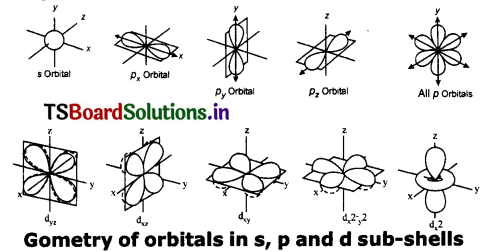Question 5.
Explain Bohr’s model of hydrogen atom and its limitations.
(OR)
Write postulates and limitations of Bohr Hydrogen atomic model.
Postulates:
(a) Electrons In an atom occupy stationary orbits of f Dced energy at different distances from the nucleus.
(b) When an electron jumps from a lower energy state to higher energy state, it absorbs energy and emits energy when such a jump occurs from a higher energy state to lower energy state.
(c) The energies of an electron in an atom can have only certain values E1, E2, E3, i.e., the energy is quantized. The states corresponding to these energies are called stationary states and the possible values of the energy are called energy levels.

Limitations
1. Bohr’s model failed to account for splitting of line spectra of hydrogen atom.
2. This model failed to account for the atomic spectra of atoms of more than one electron.

Question 6.
Do the electrons follow definite paths around the nucleus? Explain.
1. If the electron revolves around the nucleus in definite paths or orbits the exact position of the electron at various times will be known. For that, we want to know the velocity and exact position of electron.
2. Electrons are invisible to naked eye, so light of very short wavelength is required for this task.
3. This short-wavelength light interacts with the electron and disturbs the motion of the electron. Hence the position and velocity of electrons cannot be measured accurately.
4. So, it is clear that electrons do not follow definite paths In an atom.

Question 7.
Draw the diagram of electromagnetic wave.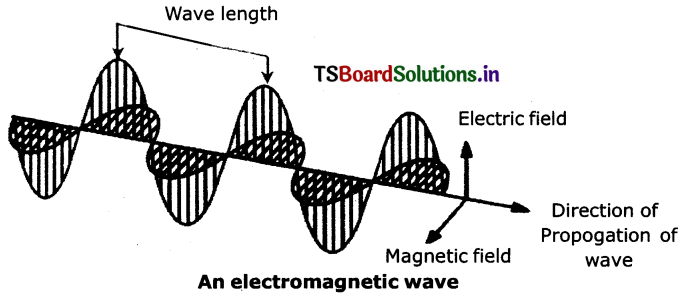Question 8.
Explain Bohr – Sommerfeld model of an atom. What is the merit of this model? What are Its limitations?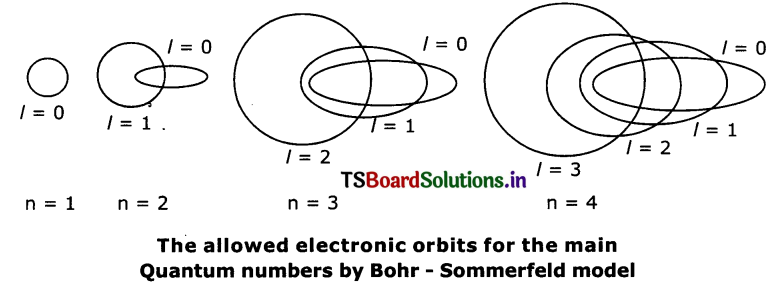1. In an attempt to account for the structure of line spectra, Sommerfeld modified Bohr’s atomic model by adding elliptical orbits.
2. While retaining the first of Bohr’s circular orbit as such, he added one elliptical orbit to Bohr’s second orbit, two elliptical orbits to Bohr’s third orbit etc.
3. The Nucleus of the atom is one of the principal foci of these elliptical orbits because periodic motion under the influence of a central force will lead to elliptical orbits with the force situated at one of the foci.
Merit: Bohr Sommerfeld’s model is successful in accounting for the fine line structure of hydrogen atomic spectra.

Limitations:
1. This model failed to account for the atomic spectra of atoms of more than one electron.
2. It did not explain Zeeman and stark effects.Question 9.
Distinguish between emission and absorption spectrum.

 Emission spectrum Absorption spectrum 1) The spectrum produced by emitted radiation is called emission spectrum. 1) The spectrum produced by absorption of radiation Is called absorption spectrum. 2) The emission spectrum contains bright lines on the dark back ground. 2) The absorption spectrum contains dark lines on the bright background. 3) The emission spectrum corresponds the radiation emitted when an excited electron returns back to the ground state. 3) The absorption spectrum corresponds the radiation absorbed in exciting an electron from lower to the higher energy levels.

Question 10.
Observe the information provided in the table about quantum numbers. Then answer the questions given below it.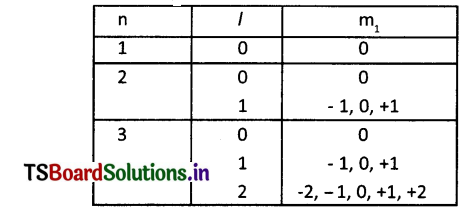(i) Write the ‘l’ value and symbol of the spherical-shaped sub-shell.
(ii) How many values that ‘m,’ takes for l=2? What are they?
(iii) Write the symbols of the orbitals for l=1 sub-shell.
(iv) What Is the shape of the sub-shell for l=2? What Is the maximum number of electrons that can occupy this sub-shell?
(i) l = 0. Its symbol is s’
(ii) m1 takes 2l+1 = 2(2)+1=5 values
They are -2, -1, 0,+1, +2
(iii) Px, Py, Pz
(iv) Double dumbelL The maximum number of electrons in this subshell are 10.

Question 11.
Observe the information and answer the following questions.

 Name of the Element Atomic Number Electronic Configuration Sodium 11 [Ne] 3s1 Magnesium 12 [Ne] 3s2 Potassium 19 [Ne] 4s1 Calcium 20 [Ne] 4s2

(1) What is valency of Magnesium?
(2) Which element has more electro-positivity?
(3) Write the elements which belongs to (third) 3rd perk,d.
(4) Write the elements which belongs to 1st Group.